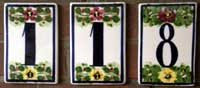## Friday, November 14, 2008

### 118

118 = 2 x 59.

118 is the smallest number that can be written as the sum of four triples, whose products are all equal: 118 = 14 + 50 + 54 = 15 + 40 + 63 = 18 + 30 + 70 = 21 + 25 + 72. The product of each triple is 37,800.

118 is the smallest three-digit composite number, all of whose other digit permutations are prime numbers: 181 and 811 are both prime.

118 is the sum of three positive cubes: 118 = 43 + 33 + 33.118 Peitho is an asteroid that was discovered in 1872.